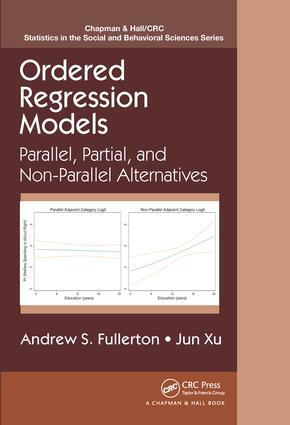# Ordered Regression Models

## Parallel, Partial, and Non-Parallel Alternatives, 1st Edition

Chapman and Hall/CRC

172 pages | 18 B/W Illus.

##### Purchasing Options:\$ = USD
Hardback: 9781466569737
pub: 2016-04-05
\$85.95
x
eBook (VitalSource) : 9780429071959
pub: 2016-04-21
from \$41.98

FREE Standard Shipping!

### Description

Estimate and Interpret Results from Ordered Regression Models

Ordered Regression Models: Parallel, Partial, and Non-Parallel Alternatives presents regression models for ordinal outcomes, which are variables that have ordered categories but unknown spacing between the categories. The book provides comprehensive coverage of the three major classes of ordered regression models (cumulative, stage, and adjacent) as well as variations based on the application of the parallel regression assumption.

The authors first introduce the three "parallel" ordered regression models before covering unconstrained partial, constrained partial, and nonparallel models. They then review existing tests for the parallel regression assumption, propose new variations of several tests, and discuss important practical concerns related to tests of the parallel regression assumption. The book also describes extensions of ordered regression models, including heterogeneous choice models, multilevel ordered models, and the Bayesian approach to ordered regression models. Some chapters include brief examples using Stata and R.

This book offers a conceptual framework for understanding ordered regression models based on the probability of interest and the application of the parallel regression assumption. It demonstrates the usefulness of numerous modeling alternatives, showing you how to select the most appropriate model given the type of ordinal outcome and restrictiveness of the parallel assumption for each variable.

Web Resource

More detailed examples are available on a supplementary website. The site also contains JAGS, R, and Stata codes to estimate the models along with syntax to reproduce the results.

### Reviews

"The book is intended to be a starter for somebody not familiar with the subject. It was written primarily for social scientists (published in the CRC Statistics in the Social and Behavioral Sciences Series) and as such, it can be read easily without any statistical pre-requisites beyond very basic Statistics and some working knowledge of logistic regression. Nevertheless, the book is certainly useful far beyond the social sciences themselves – in particular for epidemiologists, medical researchers and also statisticians of students of Statistics/Biostatistics who want to learn basic facts about ordered regression and perhaps motivate further study of this interesting field. The style of exposition is quite informal and intuitive."

~International Society for Clinical Biostatistics

Introduction

Ordinal Variables versus Ordinal Models

Brief History of Binary and Ordered Regression Models

Three Approaches to Ordered Regression Models

The Parallel Regression Assumption

A Typology of Ordered Regression Models

Asymmetrical Relationships in Partial and Nonparallel Models

Hypothesis Testing and Model Fit in Ordered Regression Models

Datasets Used in the Empirical Examples

Example: Education and Welfare Attitudes

Organization of the Book

Parallel Models

Parallel Cumulative Model

Parallel Continuation Ratio Model

Parallel Adjacent Category Model

Estimation

Conclusions

Appendix

Partial Models

Unconstrained versus Constrained Partial Models

Partial Cumulative Models

Partial Continuation Ratio Models

Partial Adjacent Category Models

Dimensionality in Partial Models

Conclusions

Appendix

Nonparallel Models

The Nonparallel Cumulative Model

The Nonparallel Continuation Ratio Model

The Nonparallel Adjacent Category Model

Practical Issues in the Estimation of Nonparallel Models

Conclusions

Appendix

Testing the Parallel Regression Assumption

Wald and LR Tests

The Score Test

The Brant Test

Additional Wald and LR Tests

Limitations of Formal Tests of the Parallel Assumption

Model Comparisons Using the AIC and the BIC

Comparing Coefficients across Cutpoint Equations

Comparing AMEs and Predicted Probabilities across Models

Conclusions

Appendix

Extensions

Heterogeneous Choice Models

Empirical Examples of Heterogeneous Choice Models

Group Comparisons Using Heterogeneous Choice Models

Introduction to Multilevel Ordered Response Regression

Bayesian Analysis of Ordered Response Regression

Empirical Examples of Bayesian Ordered Regression Models

Conclusion

References

Index

### About the Authors

Andrew S. Fullerton is an associate professor of sociology at Oklahoma State University. His primary research interests include work and occupations, social stratification, and quantitative methods. His work has been published in journals such as Social Forces, Social Problems, Sociological Methods & Research, Public Opinion Quarterly, and Social Science Research.

Jun Xu is an associate professor of sociology at Ball State University. His primary research interests include Asia and Asian Americans, social epidemiology, and statistical modeling and programing. His work has been published in journals such as Social Forces, Social Science & Medicine, Sociological Methods & Research, Social Science Research, and The Stata Journal.

### Subject Categories

##### BISAC Subject Codes/Headings:
MAT029000
MATHEMATICS / Probability & Statistics / General